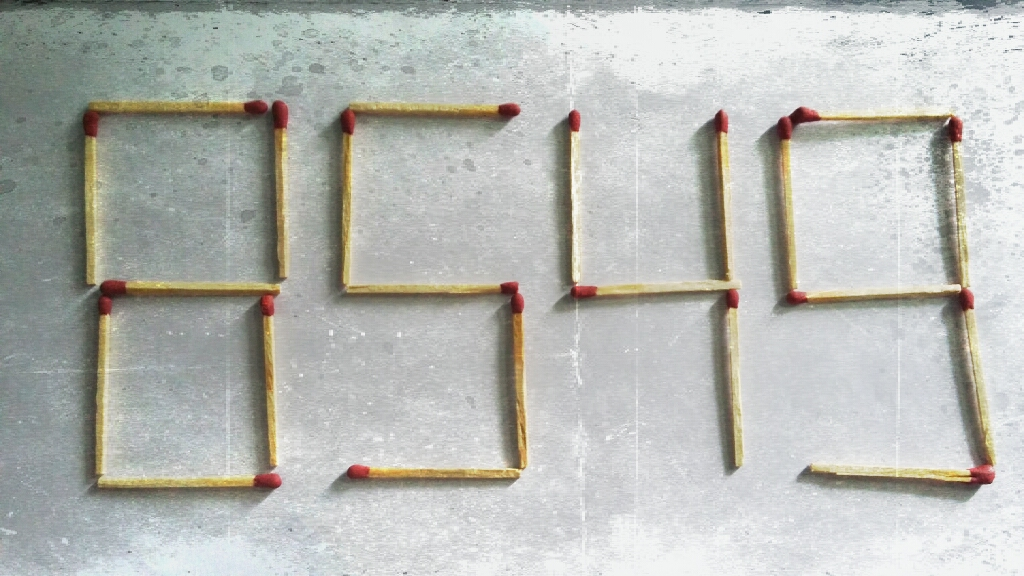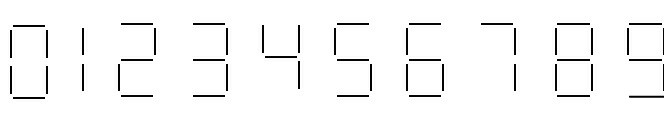# Matchstick Problem - 3

Logic Level 3The number 8549 shown in the picture above is formed by 22 matchsticks.

If you can shift exactly 2 matchsticks to another position, then what will be the $\color{#69047E} {\text{square}}$ of the $\color{#69047E} {\text {smallest}}$ integer you can make?

Details and Assumptions:

• You can't break the matchsticks.

• The digits formed by the matchsticks should be like the LED digits commonly used in calculators & LED watches. Here is an image you can follow:Check out Matchstick Problem - 4.

×